Next: Superdeformed bands in one-particle S Up: Superdeformed bands in S Previous: Signature-separation sensitive to the

## Dynamical moments and relative alignments

A mixing of two common-symmetry orbitals that approach each other at the Fermi energy creates non-converged HF solutions for certain values of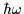as discussed in Sec. 3.4, and introduces large errors in the observables calculated in this article except, perhaps, for energies and multipole moments. Indeed, in many cases of non-converging solutions, due to the variational character of the HF equations, the total energies are almost correct, namely, they can be smoothly followed through the crossing region. However, errors in the total spins can be much larger, because the non-converged solutions correspond to almost-random mixtures of two interacting orbitals that are very close in energy but may significantly differ in spin. Then, neither the relative alignments, nor, especially, the dynamical moments, can be smoothly followed along the crossing region. Therefore, in the figures presented in this section, we removed all points corresponding to the non-converged solutions; the absence of some points was compensated for by drawing straight lines between points corresponding to the converged solutions.

In Fig. 9 are reported the dynamical moments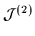=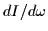, calculated for the near-yrast bands in 32S. One can see, that bands with the same intruder contents present very similar behavior, as far as the dynamical moments are concerned. It appears clearly from the figure that bands based on the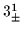and/or 30 configurations have in general (especially at high rotational frequencies) much lower dynamical moments than the magic 3232 SD band. The bands based on higher numbers of occupied intruder states (not shown in the figure), have higher values of, along with a larger distance from the yrast line.

In Fig. 10 are drawn the relative alignments,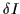=I(band)-I(SD 3232 band), of near-yrast bands in 32S calculated with respect to the magic 3232 band in the same nucleus. One can see that again the results obtained for various bands depend mainly on the numbers of occupied intruder states. It is very difficult for the nucleus to build up spin, when few intruder orbitals are occupied, and therefore one observes a lowering of the relative alignments for these bands. One may discuss these questions more clearly by introducing the relative alignments of bands in neighboring nuclei, presented in Sec. 5.

All the calculated features of SD bands in 32S seem to reflect in a very direct way the crucial role played by the intruder orbitals. Such an observation may, therefore, similarly as in other SD regions, serve as a guideline in theoretical analyses, as well as in experimental investigations of properties of SD bands in the A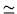30 mass region.Next: Superdeformed bands in one-particle S Up: Superdeformed bands in S Previous: Signature-separation sensitive to the
Jacek Dobaczewski
1999-07-27